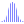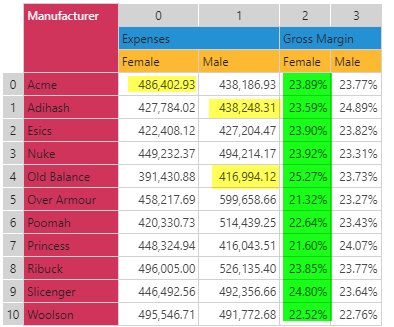#StandardDeviation (PQL)

Returns the mathematical Standard Deviation calculation across a list of numbers.

• Returned Output: Numeric
• Library: PQL \ Common \ Statistical
• Version: 2018.00.000
• Compatibility:
• Can be combined with and other PQL function throughout the application.
• It CANNOT be used with MDX or VBA functions. But it can be used on MDX-based content in other parts of the application.

#### Syntax

StandardDeviation()

or

StandardDeviation()

* Click on the function's arguments above for more details on the input values.

• The list of numbers can be typed in as a comma delimited list or inserted via an array.
• If the method is used in other parts of the application, the input parameters can be changed
• In Model, the inputs can be column names - which means to find Standard Deviation values for those columns row by row
• In Formulate, the inputs can be Data points, other functions or members
• In the dynamic querying tools (like triggers, infographics and dynamic text), the inputs can be static values or other methods
##### Different Function types
• This function is like the Excel STDEV function - calculating a Standard Deviation across a list of items.
• If you wish to find the Standard Deviation for the elements in a specified SET or LIST from a hierarchy, use this semantic function.
• Squaring the standard deviation result will return the statistical variance for the list of numbers.

#### Examples

This example simply averages the values 3 to 7 (returning 1.414)

StandardDeviation(3,4,5,6,7)This example, using the same grid above, would return the standard deviation on all the values in column 2 (numbering is zero-based). The DataSetColumn function returns an array (list) of values from column 3 in the grid above.

StandardDeviation(dataSetColumn(2))

So it would average all items 23.89%, 23.59% etc all the way through to 22.52% (1.18%)